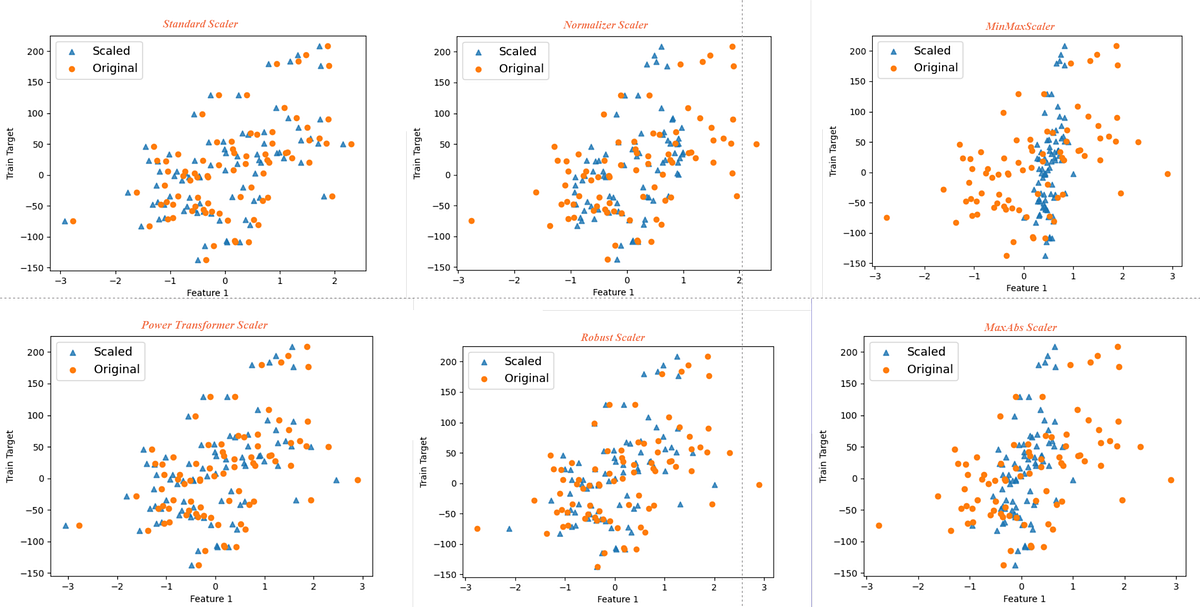# Feature Scaling — Effect Of Different Scikit-Learn Scalers: Deep DiveFeature scaling is a vital element of data preprocessing for machine learning. Implementing the right scaler is equally important. In supervised machine learning, we calculate the value of the output variable by supplying input variable values to an algorithm. Machine learning algorithm relates the input and output variable with a mathematical function.

In supervised machine learning, we calculate the value of the output variable by supplying input variable values to an algorithm. Machine learning algorithm relates the input and output variable with a mathematical function.

Output variable value = (2.4* Input Variable 1 )+ (6*Input Variable 2) + 3.5

There are a few specific assumptions behind each of the machine learning algorithms. To build an accurate model, we need to ensure that the input data meets those assumptions. In case, the data fed to machine learning algorithms do not satisfy the assumptions then prediction accuracy of the model is compromised.

Most of the supervised algorithms in sklearn require standard normally distributed input data centred around zero and have variance in the same order. If the value range from 1 to 10 for an input variable and 4000 to 700,000 for the other variable then the second input variable values will dominate and the algorithm will not be able to learn from other features correctly as expected.

In this article, I will illustrate the effect of scaling the input variables with different scalers in scikit-learn and three different regression algorithms.

In the below code, we import the packages we will be using for the analysis. We will create the test data with the help of make_regression

``````from sklearn.datasets import make_regression
import numpy as np
from sklearn.model_selection import train_test_split
from  sklearn.preprocessing import *
from sklearn.linear_model import*``````

We will use the sample size of 100 records with three independent (input) variables. Further, we will inject three outliers using the method “np.random.normal”

## Data Science With Python Training | Python Data Science Course | Intellipaat

🔵 Intellipaat Data Science with Python course: https://intellipaat.com/python-for-data-science-training/In this Data Science With Python Training video, you...

## Top 40 Python Libraries for Data Science, Data Visualization & Machine Learning

This article compiles the 38 top Python libraries for data science, data visualization & machine learning,

## Data Science Projects | Data Science | Machine Learning | Python

Practice your skills in Data Science with Python, by learning and then trying all these hands-on, interactive projects, that I have posted for you.

## Data Science Projects | Data Science | Machine Learning | Python

Practice your skills in Data Science with Python, by learning and then trying all these hands-on, interactive projects, that I have posted for you.

## Data Science Projects | Data Science | Machine Learning | Python

Practice your skills in Data Science with Python, by learning and then trying all these hands-on, interactive projects, that I have posted for you.